# Surface Area of Cylinders

In this worksheet, students calculate the surface area of cylinders given their diameter and height.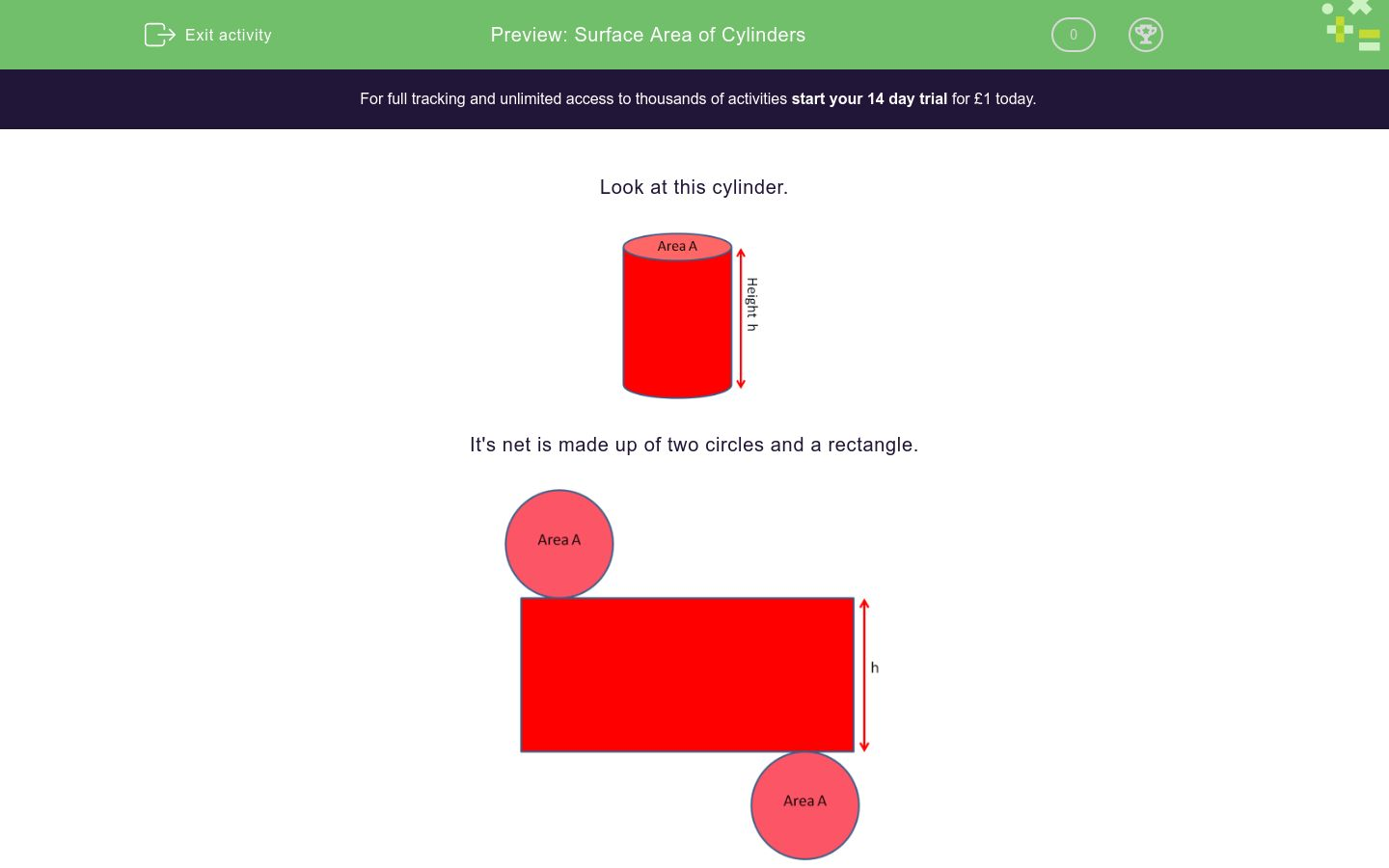Key stage:  KS 3

Curriculum topic:   Geometry and Measures

Curriculum subtopic:   Use 3D Shape Knowledge to Solve Problems

Difficulty level:### QUESTION 1 of 10

Look at this cylinder.It's net is made up of two circles and a rectangle.Remember that:

the Area of a circle of radius r = πr2

the Circumference of a circle of diameter d = πd

Look at these diagrams to see that the base of the rectangle has the same length as the circumference of the circle.So the Surface Area = 2πr2 + πdh = 2πr2 + 2πrh

Example

Find the surface area of this cylinder in cm2 to 3 significant figures.Diameter = 6 cm

Radius = 6 ÷ 2 = 3 cm

Height = 12 cm

Surface Area = 2πr2 + 2πrh  = 2 x π x 32 + 2 x π x 3 x 12 = 282.74 ≈ 283 cm2 (3 s.f.)

Find the surface area of this cylinder in cm2 to 3 significant figures.

(just write the number)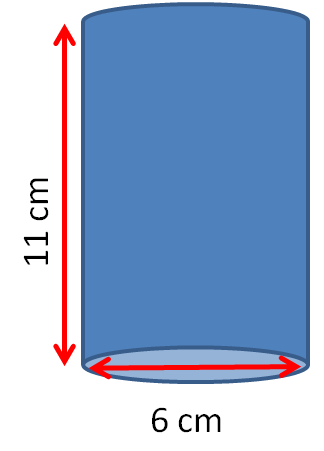Find the surface area of this cylinder in cm2 to 3 significant figures.

(just write the number)Find the surface area of this cylinder in cm2 to 3 significant figures.

(just write the number)Find the surface area of this cylinder in cm2 to 3 significant figures.

(just write the number)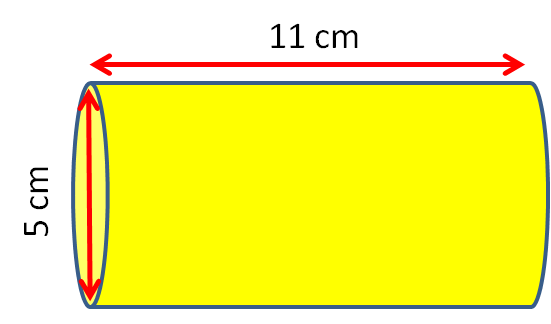Find the surface area of this cylinder in cm2 to 3 significant figures.

(just write the number)Find the surface area of this cylinder in cm2 to 3 significant figures.

(just write the number)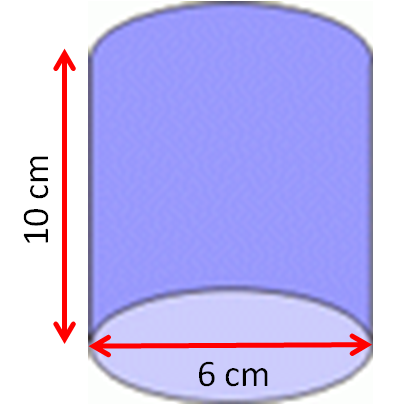Find the surface area of this cylinder in cm2 to 3 significant figures.

(just write the number)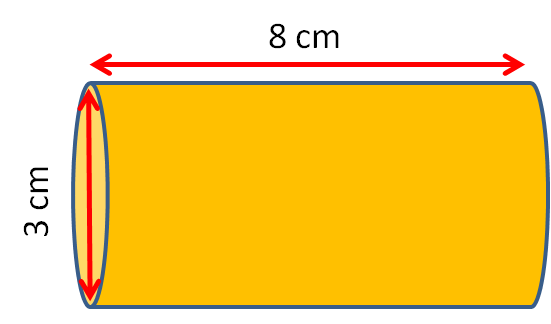Find the surface area of this cylinder in cm2 to 3 significant figures.

(just write the number)Find the surface area of this cylinder in cm2 to 3 significant figures.

(just write the number)Find the surface area of this cylinder in cm2 to 3 significant figures.

(just write the number)• Question 1

Find the surface area of this cylinder in cm2 to 3 significant figures.

(just write the number)264
EDDIE SAYS
(2 x area of the circle at the end) + (The area of the circle x the height of the cylinder) (2 × π × r²) + (2 × π × r × h) (2 × π × 3²) + (2 × π × 3 × 11)
• Question 2

Find the surface area of this cylinder in cm2 to 3 significant figures.

(just write the number)352
EDDIE SAYS
(2 x area of the circle at the end) + (The area of the circle x the height of the cylinder) (2 × π × r²) + (2 × π × r × h) (2 × π × 4²) + (2 × π × 4 × 10)
• Question 3

Find the surface area of this cylinder in cm2 to 3 significant figures.

(just write the number)363
EDDIE SAYS
(2 x area of the circle at the end) + (The area of the circle x the height of the cylinder) (2 × π × r²) + (2 × π × r × h) (2 × π × 5.5²) + (2 × π × 5.5 × 5)
• Question 4

Find the surface area of this cylinder in cm2 to 3 significant figures.

(just write the number)212
EDDIE SAYS
(2 x area of the circle at the end) + (The area of the circle x the height of the cylinder) (2 × π × r²) + (2 × π × r × h) (2 × π × 2.5²) + (2 × π × 2.5 × 11)
• Question 5

Find the surface area of this cylinder in cm2 to 3 significant figures.

(just write the number)730
EDDIE SAYS
(2 x area of the circle at the end) + (The area of the circle x the height of the cylinder) (2 × π × r²) + (2 × π × r × h) (2 × π × 7.5²) + (2 × π × 7.5 × 8)
• Question 6

Find the surface area of this cylinder in cm2 to 3 significant figures.

(just write the number)245
EDDIE SAYS
(2 x area of the circle at the end) + (The area of the circle x the height of the cylinder) (2 × π × r²) + (2 × π × r × h) (2 × π × 3²) + (2 × π × 3 × 10)
• Question 7

Find the surface area of this cylinder in cm2 to 3 significant figures.

(just write the number)89.5
EDDIE SAYS
(2 x area of the circle at the end) + (The area of the circle x the height of the cylinder) (2 × π × r²) + (2 × π × r × h) (2 × π × 1.5²) + (2 × π × 1.5 × 8)
• Question 8

Find the surface area of this cylinder in cm2 to 3 significant figures.

(just write the number)138
EDDIE SAYS
(2 x area of the circle at the end) + (The area of the circle x the height of the cylinder) (2 × π × r²) + (2 × π × r × h) (2 × π × 2²) + (2 × π × 2 × 9)
• Question 9

Find the surface area of this cylinder in cm2 to 3 significant figures.

(just write the number)881
EDDIE SAYS
(2 x area of the circle at the end) + (The area of the circle x the height of the cylinder) (2 × π × r²) + (2 × π × r × h) (2 × π × 8.5²) + (2 × π × 8.5 × 8)
• Question 10

Find the surface area of this cylinder in cm2 to 3 significant figures.

(just write the number)442
EDDIE SAYS
(2 x area of the circle at the end) + (The area of the circle x the height of the cylinder) (2 × π × r²) + (2 × π × r × h) (2 × π × 6.25²) + (2 × π × 6.25 × 5)
---- OR ----

Sign up for a £1 trial so you can track and measure your child's progress on this activity.

### What is EdPlace?

We're your National Curriculum aligned online education content provider helping each child succeed in English, maths and science from year 1 to GCSE. With an EdPlace account you’ll be able to track and measure progress, helping each child achieve their best. We build confidence and attainment by personalising each child’s learning at a level that suits them.

Get started